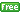www.gllamm.org

## Why do I get the message "weight variables not found?" when I specify the weight() option?

 Title Specifying frequency weights in gllamm Author Minjeong Jeon, University of California, Berkeley Date July 2012

This problem has to do with specifying weight variables in the `weight(stubname)` option. For example, `weight(wt)` specifies that variables `wt1`, `wt2`, etc. contain frequency weights. `wt` is just the stub of the variable names. The variable names must begin with the same stub followed by a number. The numbers in the variable names (`wt1`, `wt2`, etc.) determine to which level each weight applies. For instance, `wt1` is the frequency weight at level 1 and `wt2` is the frequency weight at level 2, etc. You do not need weight variables at all levels, but for the levels with no weight variables, `gllamm` assumes the weights are equal to 1.

For example, suppose you have a three-level of data, but you have only `myweight2` in the dataset. Specify `myweight` in the `weight()` option in the `gllamm` command:

```gllamm ... , weight(myweight)
```
It is very important to use `myweight` (not `myweight2`) inside the parentheses for `weight`. Since there are no `myweight1` and `myweight3` in the data set, `gllamm` assumes that frequency weights at level 1 and 3 (`myweight1` and `myweight3`) are equal to 1.

### Examples and documentation

• Description of `weight()` option on p.22-23, and examples in Sections 3.2.2, 4.1.1-4.1.2, 8.3.1-8.3.2, 8.4, and 9.3 of Rabe-Hesketh, S., Skrondal, A. and Pickles, A. (2004). GLLAMM Manual.U.C. Berkeley Division of Biostatistics Working Paper Series. Working Paper 160.
• Skrondal, A. and Rabe-Hesketh, S. (2004). Generalized Latent Variable Modeling: Multilevel, Longitudinal and Structural Equation Models. Chapman & Hall/CRC.
• Exercises 10.3, 10.7, 14.5, and 16.11 and p.929-930 in the book Rabe-Hesketh, S. and Skrondal, A. (2012). Multilevel and Longitudinal Modeling Using Stata (Third Edition). Volume II: Categorical Responses, Counts, and Survival. College Station, TX: Stata Press.